﻿ 关于电缆卷绕过程的状态监测研究 Study on State Monitoring of Cable Winding Process

Journal of Sensor Technology and Application
Vol. 07  No. 03 ( 2019 ), Article ID: 31208 , 10 pages
10.12677/JSTA.2019.73012

Study on State Monitoring of Cable Winding Process

Chao Cheng, Wei Fan, Jingyi Zhang

College of Automation, Nanjing University of Science and Technology, Nanjing JiangsuReceived: Jun. 18th, 2019; accepted: Jul. 3rd, 2019; published: Jul. 10th, 2019ABSTRACT

Cable winding is the final step of cable production, but in practice, there is no safe and efficient detection scheme. Therefore, if the cable is not neatly arranged during winding, the system cannot provide timely feedback. In order to solve this problem, a method of least square circle fitting based on radius constraint is proposed to realize the measurement of cable winding. At the same time, the influence of various factors on the fitting accuracy is analyzed in detail, and the simulation calculation is carried out.

Keywords:Cable Status Detection, Least Square Method, Radius Constraint, The Error Analysis1. 引言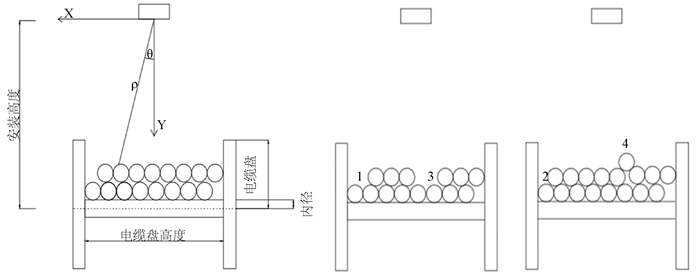(a) (b) (c)

Figure 1. Alignment of cables. (a) In alignment; (b) Misaligned1; (c) Misaligned 2

2. 国内外研究现状

3. 状态检测的算法设计

3.1. 基本原理

$x=\rho \cdot \mathrm{sin}\theta \text{\hspace{0.17em}}\text{\hspace{0.17em}}\text{\hspace{0.17em}}\text{\hspace{0.17em}}\text{}y=\rho \cdot \mathrm{cos}\theta$ (1)

3.2. 算法设计

3.2.1. 数据预处理

3.2.2. 确定边界位置

${x}_{L}=\frac{1}{N}\underset{i=1}{\overset{N}{\sum }}{x}_{i}{x}_{R}=\frac{1}{N}\underset{j=1}{\overset{N}{\sum }}{x}_{j}$ (2)

3.2.3. 确定电缆位置

${\left(x-{x}_{0}\right)}^{2}+{\left(y-{y}_{0}\right)}^{2}={r}_{0}^{2}$ (3)

$\underset{i=1}{\overset{N}{\sum }}{\delta }_{i}^{2}={\left[{\left({x}_{i}-{x}_{0}\right)}^{2}+{\left({y}_{i}-{y}_{0}\right)}^{2}-{r}_{0}^{2}\right]}^{2}$ (4)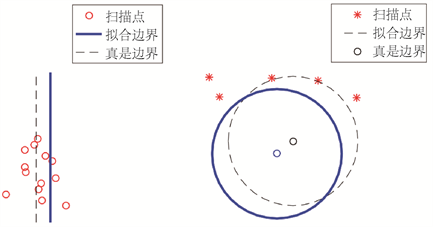(a) (b)

Figure 2. The fitting process. (a) The process of fitting a line; (b) The process of fitting a circle

4. 拟合精度影响因素及扫描次数确定

4.1. 模型建立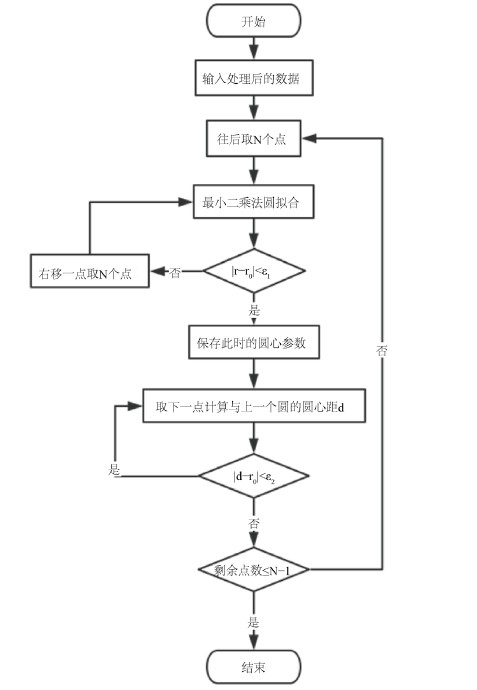Figure 3. Flow chart for determining the center of circles

4.2. 边界拟合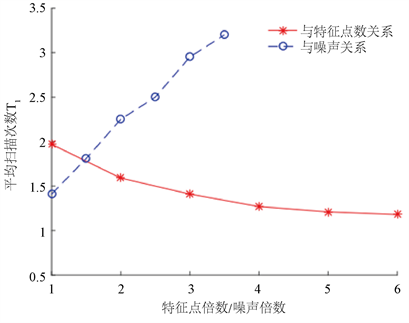Figure 4. Boundary fitting error

4.3. 电缆圆心拟合

5. 相关要求

5.1. 电缆规格

$\frac{r}{\sqrt{{\left(\frac{D}{2}-r\right)}^{2}+{\left(h-r\right)}^{2}}}=\mathrm{sin}1.5\theta$ (5)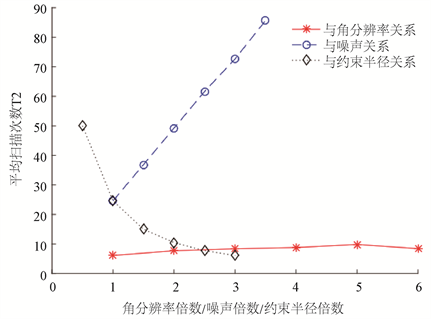Figure 5. Fitting error of cable center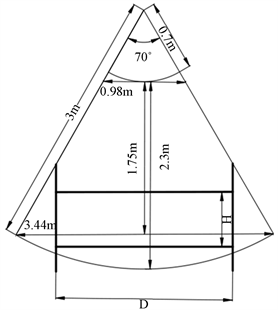Figure 6. Schematic diagram of sensor detection range

5.2. 卷绕速度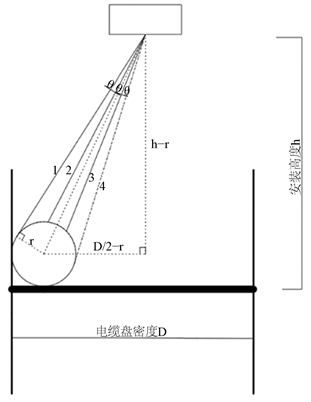Figure 7. Determination of cable specifications

$t>\frac{{T}_{2}}{f}$ (6)

5.3. 电缆盘规格

LMS400的检测范围如图6所示，考虑传感器的扫描范围充分覆盖电缆盘及电缆，所以适当缩小有效检测范围以确保检测的准确性。以角度范围60˚，长度范围0.8 m至2.9 m为例进行计算，实际应用中可对检测范围的取值做相应调整。

$1\right)\text{\hspace{0.17em}}\text{\hspace{0.17em}}0\text{\hspace{0.17em}}\text{m} (7)

$2\right)\text{\hspace{0.17em}}\text{\hspace{0.17em}}0.92\text{\hspace{0.17em}}\text{m} (8)

5.4. 安装要求

1) 电缆卷盘内宽最大值

$f\left(h\right)=\left\{\begin{array}{l}\frac{2\sqrt{3}}{3}h\text{\hspace{0.17em}}\text{\hspace{0.17em}}\text{\hspace{0.17em}}\text{\hspace{0.17em}}\text{\hspace{0.17em}}\text{\hspace{0.17em}}\text{\hspace{0.17em}}\text{\hspace{0.17em}}\text{\hspace{0.17em}}\text{\hspace{0.17em}}\text{\hspace{0.17em}}\text{\hspace{0.17em}}\text{\hspace{0.17em}}\text{\hspace{0.17em}}\text{\hspace{0.17em}}0.8\text{\hspace{0.17em}}\text{m} (9)

2) 当 $0.8\text{\hspace{0.17em}}\text{m}

$f\left(h-H\right)=2\sqrt{3}\left(h-H\right)/3$ (10)

$g\left(h\right)=\left[f\left(h-H\right)-D\right]/5=\sqrt{3}\left(h-H\right)/3-D/2$ (11)

3) 当 $2.511\text{\hspace{0.17em}}\text{m}\le h<2.9\text{\hspace{0.17em}}\text{m}$$2.511\text{\hspace{0.17em}}\text{m}<\left(h-H\right)<2.9\text{\hspace{0.17em}}\text{m}$

$f\left(h\right)=2\sqrt{8.41-{h}^{2}}$ (12)

$g\left(h\right)=\left[f\left(h\right)-D\right]/2=\sqrt{8.41-{h}^{2}}-D/2$ (13)

4) 当 $0.8\text{\hspace{0.17em}}\text{m}<\left(h-H\right)<2.511\text{\hspace{0.17em}}\text{m}$$2.511\text{\hspace{0.17em}}\text{m}\le h<2.9\text{\hspace{0.17em}}\text{m}$

$f\left(h-H\right)=2\sqrt{3}\left(h-H\right)/3$ (14)

$f\left(h\right)=2\sqrt{8.41-{h}^{2}}$ (15)

$g\left(h\right)=\left(\mathrm{min}\left\{f\left(h-H\right),f\left(h\right)\right\}-D\right)/2$ (16)

6. 总结

Study on State Monitoring of Cable Winding Process[J]. 传感器技术与应用, 2019, 07(03): 104-113. https://doi.org/10.12677/JSTA.2019.73012

1. 1. 王江平, 田亮, 付忠文, 李钦彬. 井下设备电缆卷缆装置的改装设计[J]. 煤矿机械, 2011, 32(9): 20-21.

2. 2. Arai, M. and Hirose, S. (2007) Improved Reel Mechanism to Wind a Cable Uniformly in Spherical Trailer. 2007 IEEE In-ternational Workshop on Safety, Security and Rescue Robotics, Rome, 27-29 September 2007, 1-6. https://doi.org/10.1109/SSRR.2007.4381257

3. 3. 杨洋, 李柯, 杨学敬, 俞友爱, 江涛, 顾菁宇. 电缆卷绕装置以及车载移动式检测设备[P]. 中国. 201710170091.7, 2017-03-21.

4. 4. 吴笛, 倪晋平, 陈丁, 李海, 孟炳全. 360˚激光测距的可移动三维建模方法[J]. 测试技术学报, 2019, 33(1): 14-18.

5. 5. 周封, 杨超, 王晨光, 等. 基于随机Hough变换的复杂条件下圆检测和数目辨识[J]. 仪器仪表学报, 2013, 34(3): 623-624.

6. 6. 王新, 张元东, 王莉. 一种随机Hough变换检测圆的优化方法[J]. 测控技术, 2016, 35(6): 112-114.

7. 7. 刘珂, 周富强, 张广军. 半径约束最小二乘圆拟合方法及其误差分析[J]. 光电子•激光, 2006, 17(5): 604-607.

8. 8. 朱嘉, 李醒飞, 谭文斌, 等. 基于圆心约束最小二乘圆拟合的短弧测量[J]. 光学精密工程, 2009, 17(10): 2487-2489.

9. 9. 安培源. 基于机器视觉的多圆工件检测技术研究[D]: [硕士学位论文]. 杭州: 浙江理工大学, 2018.

10. 10. Xu, W., Chen, W. and Liang, Y. (2018) Feasibility Study on the Least Square Method for Fitting Non-Gaussian Noise Data. Physica A: Statistical Mechanics and Its Applications, 492, 1917-1930. https://doi.org/10.1016/j.physa.2017.11.108